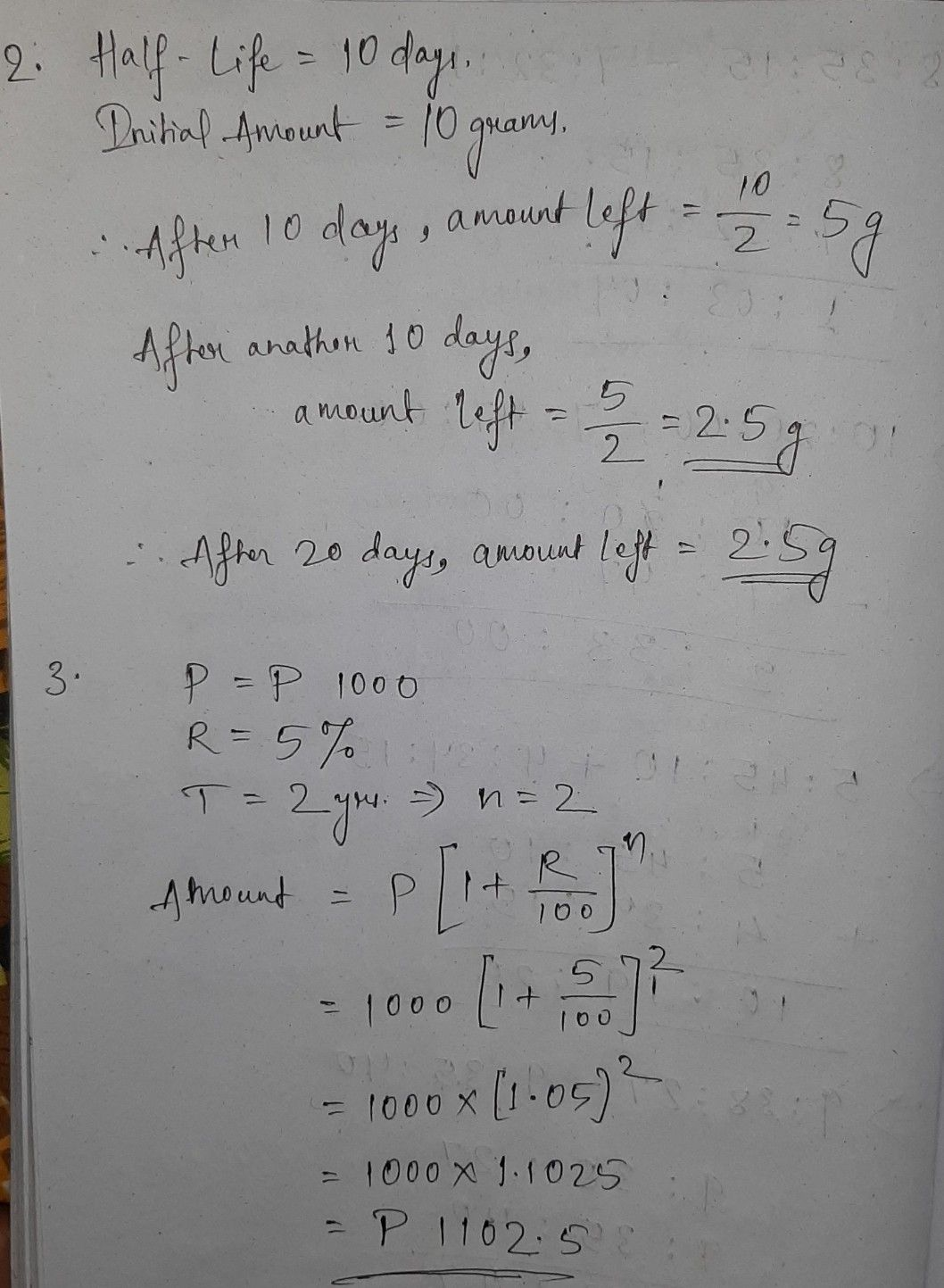Symbol
Problem$2$ 2. The $half-lif_{e}$ of $a$ radioactive substance is $10$ days and there are $10$ grams initially. Determine the amount of substance left after $20$ $days$ $3.$ Eunice deposited $P1,000$ in $a$ bank that pays $5%$ compound interest annually. How much money will she save after $2y$ years?
10th-13th grade
Trigonometry
Search count: 112
Question content
Solving problems Involving Exponential Functions,Exponential Equations and Exponential Inequalities
SolutionQanda teacher - BhanuStudent
thankyouQanda teacher - Bhanu
welcome